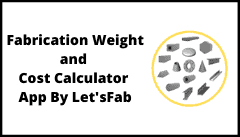## Fabrication Weight and Cost Calculator App by Let’sFab# Fabrication Weight and Cost Calculator App by Let’sFab

Fabrication Weight and Cost Calculator App is used to Calculate Fabrication Weight and Cost of most widely used Fabrication Shapes and Steel Sections or Steel Structures Such as Plate, Pipe, Circle, Round, Tube, Shell, Dish End, Cone, Flange, Angle, Channel, Beam, Tee Section, Square Tube, Square Bar, Triangular Bar, Hexagonal Bar, etc.

This Calculator is also called as Metal Weight Calculator, Engineering Weight calculator, Section Weight Calculator, Steel Weight Calculator, Steel Section Weight Calculator, Steel Structure Weight Calculator, Metal Cost Calculator, Steel Cost Calculator, Metal Cost Estimator, Steel Cost Estimator and so on. All Dimensions required in Metric System or MM.

## In This App Following Calculator are available :

1. Plate Weight Calculator.
2. Circle Weight Calculator or Round Bar Weight Calculator.
3. Pipe Weight Calculator or Shell Weight Calculator or Tube Weight Calculator.
4. Cone Weight Calculator or Full Cone Weight Calculator or Concentric Cone Weight Calculator.
5. Truncated Cone Weight Calculator or half Cone Weight Calculator.
6. Dish End Weight Calculator or Vessel Heads Weight Calculator or End Cap Weight Calculator.
7. Channel Weight Calculator or C Section Weight Calculator.
8. Beam Weight Calculator or I Section Weight Calculator.
9. Angle Weight Calculator or L section Weight Calculator.
10. Square Tube Weight Calculator or Hallow Tube Weight Calculator or Hallow Section Weight Calculator.
11. Tee Weight Calculator or T Section Weight Calculator.
12. Square Bar Weight Calculator or Square Section Weight Calculator.
13. Hex-bar Weight Calculator or Hex Section Weight calculator.
14. Triangular Bar Weight Calculator or Triangular Section Weight Calculator.
15. Flange Weight Calculator.

Fabrication Weight and Cost Calculator is build to calculate fabrication weight and cost. It is useful for various shapes which are widely used in fabrication Industry, Construction Industry, Equipment Manufacturing Industry, Steel Structural Fabrication Industry, Process Equipment Manufacturing Industry, Heavy Equipment Manufacturing Industry.

## How To Use This Application:

In this tool Home Page has given different Options to Section For Weight Calculations. You have to Select any Calculator as Listed in the list. In this Calculator Plate, Round Bar, Pipe, Cone, Truncated Cone, Dish Ends, Channel, Angle, Beam, Square bar, Hex bar, Triangular Bar, Tee Sections and Flange etc. options are available.

After selecting your tools it will opens input data page. Then you have to enter all inputs required for this weight calculator in input fields. When you Complete filling all Data to input Fields then Click on Calculate Button. It will show you output results as Unit Weight and Total Weight. if you have missed any data input it will prompt you to check your input fields. then fill all data as per requirement and then Click on Calculate button.

You Can Select Material Sp. Gravity by Clicking on Material Selection Button. It will open Material Selection List you can directly enter Sp. Gravity, if your Material is not in to List.

It Supports All Commonly used Materials such as Carbon steel or Mild Steel, Stainless Steel, Aluminium, Brass, Bronze, Lead, Copper, Cast Iron etc. if your Material is not available you can manual enter specific Gravity to calculate weight of your material.

Fabrication Weight and Cost calculator is useful for professional work in the field of fabrication of Process equipment. It is more useful in Pressure vessel, Heat Exchanges, Storage tank, Piping, Structures or which are in engineering services.